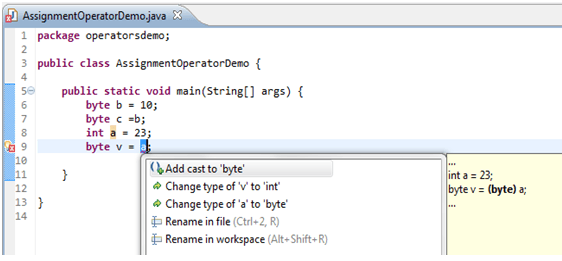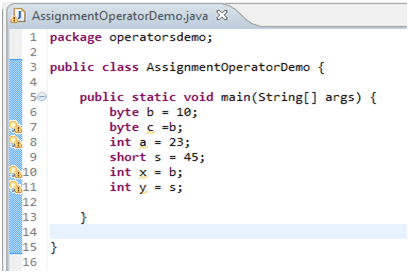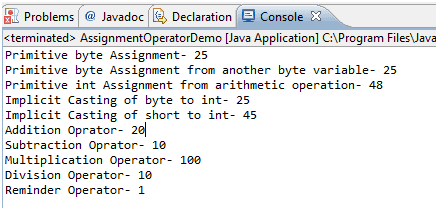## Description

Assigning a value to a variable seems straightforward enough; you simply assign the stuff on the right side of the '= 'to the variable on the left. Below statement 1 assigning value 10 to variable x and statement 2 is creating String object called name and assigning value "Amit" to it.

```Statement 1:     x =10;
Statement 2:   String name = new String ("Amit");
```

Assignment can be of various types. Let’s discuss each in detail.

## Primitive Assignment :

The equal (=) sign is used for assigning a value to a variable. We can assign a primitive variable using a literal or the result of an expression.

```int x = 7; // literal assignment
int y = x + 2; // assignment with an expression
int z = x * y; // assignment with an expression with literal
```

## Primitive Casting

Casting lets you convert primitive values from one type to another. We need to provide casting when we are trying to assign higher precision primitive to lower precision primitive for example If we try to assign int variable (which is in range of byte variable) to byte variable then compiler will throw exception called "possible loss of precision". Eclipse IDE will suggest the solution as well as shown below. To avoid such problem we should use type casting which will instruct compiler for type conversion.

```byte v = (byte) a;
```For cases where we try to assign smaller container variable to larger container variables we do not need of explicit casting. Compiler will take care of those type conversions. For example we can assign byte variable or short variable to int without any explicit casting.## Assigning Literal that is too large for a variable

When we try to assign variable value which is too large (or out of range ) for primitive variable then compiler will throw exception “possible loss of precision” if we try to provide explicit cast then compiler will accept it but narrowed down the value using two’s compliment method. Let’s take example of byte which has 8 bit storage space and range -128 to 127. In below program we are trying to assign 129 literal value to byte primitive type which is out of range for byte so compiler converted it to -127 using two’s compliment method. Refer link for two’s complement calculation (http://en.wikipedia.org/wiki/Two's_complement)

1. public class ExplicitCastingDemo {
2.     public static void main(String[] args) {
3.         byte b = (byte)129;
4.         System.out.println("Value of b= " + b);
5.     }
6. }

## Output## Reference variable assignment

We can assign newly created object to object reference variable as below

```String s = new String(“Amit”);
Employee e = New Employee();
```

First line will do following things,

• Makes a reference variable named s of type String
• Creates a new String object on the heap memory
• Assigns the newly created String object to the reference variable s

You can also assign null to an object reference variable, which simply means the variable is not referring to any object. The below statement creates space for the Employee reference variable (the bit holder for a reference value), but doesn't create an actual Employee object.

```Employee a = null;
```

## Compound Assignment Operators

Some time we need to modify same variable value and reassigned it to same reference variable. Java allows you to combine assignment and addition operators using a shorthand operator. For example, the preceding statement can be written as:

```i +=8; //This is same as i = i+8;
```

The += is called the addition assignment operator. Other shorthand operators are shown below table

Operator Name Example Equivalent
-= Subtraction assignment j-=10; j=j-10;
*= Multiplication assignment k*=2; k=k*2;
/= Division assignment x/=10; x=x/10;
%= Remainder assignment a%=4; a=a%4;

Below is the sample program explaining assignment operators:

1. public class AssignmentOperatorDemo {
2.     public static void main(String[] args) {
3.         //Literal Primitive Assignment
4.         byte b = 25;
5.         System.out.println("Primitive byte Assignment- "+ b);
6.         //Assigning one primitive to another primitive
7.         byte c =b;
8.         System.out.println("Primitive byte Assignment from another byte variable- "+ c);
9.         //Literal assignment based on arithmetic operation
10.         int a = 23+b;
11.         System.out.println("Primitive int Assignment from arithmetic operation- "+ a);
12.         //Implicit Casting of variable x and y
13.         short s = 45;
14.         int x = b;
15.         int y = s;
16.         System.out.println("Implicit Casting of byte to int- "+ x);
17.         System.out.println("Implicit Casting of short to int- "+ y);
18.         //Short-Hand Assignment Operators
19.         int i = 10;
20.         i+=10;
22.         i-=10;
23.         System.out.println("Subtraction Oprator- "+ i);
24.         i*=10;
25.         System.out.println("Multiplication Operator- " + i);
26.         i/=10;
27.         System.out.println("Division Operator- " + i);
28.         i%=3;
29.         System.out.println("Reminder Operator- " + i);
30.     }
31. }

## Output## Summary

• Assigning a value to can be straight forward or casting.
• If we assign value which is out of range of variable type then 2’s compliment is assigned.
• Java supports shortcut/compound assignment operator.

Assignment Operator

Arithmetic Operator

Conditional Operator

Logical Operator

source:http://www.w3resource.com/java-tutorial/java-assignment-operators.php